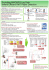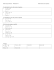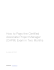# mgarch

## Transcription

mgarch
```Multivariate GARCH models.
Financial market volatility moves together over time across assets and markets. Recognizing this commonality through a multivariate modeling framework
leads to obvious gains in eﬃciency.
Following Bollerslev et al (1986) consider a multivariate extension of the
GARCH(p,q) as follows:
Consider a system of n regression equations,
yt = μ + ut
vech(Ht ) = C +
p
X
Ai vech(ut−i u0t−i ) +
i=1
q
X
Bi vech(Ht−i )
i=1
where ut |It−1 ~N(0,Ht )
In this formulation, Ht = E(ut u0t |It−1 ) is the n × n conditional variance
matrix associated with the error vector u0t and vech(Ht ) denotes the (n(n+1))/2
× 1 vector of all the unique elements of Ht obtained by stacking the lower
triangle of Ht 1 .
Also
• μ is n × 1
• C is (n(n + 1))/2 × 1
• A1 , A2 , ...Ap , B1 , ...Bq are (n(n + 1))/2 × (n(n + 1))/2
Example: A Bivariate GARCH(1,1)
Since most empirical applications of the model have restricted attention to
multivariate GARCH(1,1) systems. We first consider the easiest example is the
simple bivariate process which depends on its conditional variance covariance
matrix (Ht ).
1 In
general if
a c
b d
Then
⎡
A=
⎤
a
⎢ b ⎥
⎥
vech(A) = ⎢
⎣ c ⎦
d
For a symmetric matrix
a c
B=
c d n×n
is standard ⎡to write
⎤ the vector using either the lower or the upper triangular information
a
vech(B) = ⎣ c ⎦
d (n×(n+1)/2)×1
1
∙
xt
wt
¸
=
∙
μx
μw
¸
+
∙
εt
νt
¸
where
⎤ ⎡
⎤⎡
⎤
⎤
⎡
a1 a2 a3
(εt−1 )2
Vt−1 (xt )
cx
⎣ COVt−1 (xt wt ) ⎦ = ⎣ cxw ⎦ + ⎣ a4 a5 a6 ⎦ ⎣ (εt−1 ν t−1 ) ⎦
Vt−1 (wt )
(ν t−1 )2
cw
a7 a8 a9
⎡
⎤⎡
⎤
b1 b2 b3
Vt−2 (xt−1 )
+ ⎣ b4 b5 b6 ⎦ ⎣ COVt−2 (xt−1 wt−1 ) ⎦
Vt−2 (wt−1 )
b7 b8 b9
⎡
Estimation of this model may be obtained by ML using numerical procedures.
Two main problems with the estimation of these type of models should be
apparent:
1. As its stands this model is very general, requiring a large number of parameters to be estimated. The ”simplest” general model has 23 parameters.
2. We should ensure Ht to be positive definite. (see Bera and Higgins).
Various simplifications have been suggested to account for (1) and (2).
A Diagonal Vech Parameterization
(Bollerslev, Engle and Wooldridge (1982))
A natural simplification is to assume that each covariance depends only on
its own past values and innovations, i.e., that Ai and Bi are diagonal. Then Each
element of Ht follows an univariate GARCH model driven by the corresponding
cross product ut u0t . The implied conditional covariance matrix is always positive
definite if the matrices of parameters
∙
¸ ∙
¸ ∙
¸
cx cxw
a1 a5
b1 b5
,
,
cxw cw
a5 a9
b5 b9
are all positive definite. This can be ensured by doing simple Cholesky transformations to each of these matrices.
(Engle and Kroner(1995))
An alternative to the diagonal vech parameterization is to achieve a positive
definite covariance matrix is the quadratic parameterization
2
Ht = C 0 C + A0ut−1 u0t−1 A + B 0 Ht−1 B.
where C is a lower triangular with n(n + 1)/2 parameters and A and B are
square matrices with n2 parameters each. Weak restrictions on A and B guarantee that the Ht is always positive definite.
A Constant-Correlation Specification,
(Bollerslev(1990))
This specification is similar to the diagonal specification, but imposes the
restriction that the correlation between the assets is constant.
p
COVt−1 (xt wt ) = ρ Vt−1 (xt )Vt−1 (wt )
Where ρ is also estimated with the rest of the parameter set. The conditions to
get a positive definite matrix are as in the diagonal case.
Stationarity and Co-persistence
Stationarity
The conditions for stationarity and moment convergence for the multivariate
case are similar to those discussed in the univariate case. Specially, for the
multivariate vech GARCH(1,1) model defined above, the minimum square error
forecast for vech(Ht ) as of time s < t takes the form
t−s−1
X
(A1 + B1 )k ]C + (A1 + B1 )t−s vech(Hs )
Es (vech(Ht )) = [
k=0
0
where (A1 + B1 ) is equal to the identity matrix by definition.
Let V ΛV −1 denote the Jordan decomposition of the matrix A1 + B1 , so that
(A1 +B1 )t−s = V Λt−s V −1 . Thus, Es (vech(Ht )) converges to the unconditional
covariance matrix (I − A1 − B1 )−1 C , for t → ∞, if and only if the absolute
value of the largest eigen value of A1 + B1 is strictly less than one.
Results for other multivariate formulations are scarce, a possible exception is
the constant conditional correlations parameterization where the conditions for
the model to be covariance stationary are simply determined by the conditions
of each of the univariate conditional variances.
Co-Persistence in Variance
The empirical estimates for univariate and multivariate ARCH models often
indicate a high degree of persistence in the forecast moments of the conditional
variances, i.e. Es (Ht )ii , i = 1, 2, ....N , for t → ∞. At the same time, the
3
commonality in volatility movements suggest that this persistence may be common across diﬀerent series. More formally Bollerslev and Engle (1993) define
the multivariate ARCH process to be co-persistent in variance if at least one
of the elements in Es (Ht ) is non-convergent for increasing forecasts, t − s, but
there exists a linear combination γ 0 εt , such that for every forecast origin s, the
forecasts of the corresponding future conditional variances Es (γ 0 Ht γ) converge
to a finite limit, independent of time s information. The conditions for this to
occur are presented in Bollerslev and Engle (1993) and are similar to the conditions for co-integration in the mean as developed by Engle and Granger (1987).
Multivariate GARCH-M models
.
Engle and Bollerslev (1986) consider a multivariate extension of the gARCHm as follows:
Consider a system of n regression equations,
yt = BXt + Dvech(Ht ) + ut
p
X
vech(Ht ) = C +
Ai vech(ut−i u0t−i )
i=1
where ut |It−1 ˜N (0, Ht )
+
q
X
Bi vech(Ht−i )
i=1
Where
• B is n × k
• D is n × (n(n + 1))/2
• C is (n(n + 1))/2 × 1
• A1 , A2 , ...Ap , B1 , ....Bq are (n(n + 1))/2 × (n(n + 1))/2
A Bivariate GARCH-M(1,1) model
The easiest example is the simple bivariate process which depends on its
conditional variance covariance matrix (Ht )
∙
xt
wt
¸
=
∙
μx
μw
¸
+
∙
d1
d4
d2
d5
d3
d6
where
4
¸
⎡
⎤
¸
∙
Vt−1 (xt )
⎣ COVt−1 (xt wt ) ⎦ + εt
νt
Vt−1 (wt )
⎡
⎤ ⎡
⎤⎡
⎤
⎤
⎡
Vt−1 (xt )
c1 c2 c3
(εt−1 )2
cx
⎣ COVt−1 (xt wt ) ⎦ = ⎣ cxw ⎦ + ⎣ c4 c5 c6 ⎦ ⎣ (εt−1 ν t−1 ) ⎦
Vt−1 (wt )
(ν t−1 )2
cw
c4 c5 c6
⎡
⎤⎡
⎤
b1 b2 b3
Vt−2 (xt−1 )
+ ⎣ b4 b5 b6 ⎦ ⎣ COVt−2 (xt−1 wt−1 ) ⎦
Vt−2 (wt−1 )
b4 b5 b6
Estimation
The estimation of all the models presented above is carried out using conditional maximum likelihood estimation. The conditional log likelihood function
for a single observation can be written as
Lt (θ) = −(n/2) log(2π) − (1/2) log(Ht (θ)) − (1/2)ut (θ)0 Ht−1 (θ)ut (θ)
where θ represents a vector of parameters, n represents number of equations
and t represents time.
Then conditional on initial values for u0 and H0 , the likelihood function for
the sample 1, .., T can be written as
L(θ) =
T
X
Lt (θ)
t=1
The maximization is usually achieved through numerical methods Notice that
the model is highly non-linear and very unstable.
An application of the MGARCH-M model : Testing The CAPM
General issues
Three main diﬃculties associated with testing the CAPM
1. The CAPM is a statement about the relationships between ex ante risk
premiums and betas, both of which there are not directly observable. This
problem is usually dealt with by assuming that investors form rational
expectations: the realized return on assets are drawings from the ex ante
probability distribution of returns on those assets.
2. The Roll- critique; many assets are not measurable (human capital etc.)
so tests of the CAPM have to be based on proxies for the market portfolio
which only includes (a subset of) traded assets.
5
3. The CAPM is a single-period model. However, in order to test the model
time series are frequently used. This is only a valid procedure if both risk
premia and betas are stationary.
The Unconditional CAPM a short review of the literature.
Markowitz(1959) laid the ground work for the CAPM. He cast the investor’s
portfolio selection problem in terms of the expected return and variance of the
return. He argued that investors would optimally hold a mean-variance eﬃcient
portfolio. Sharpe and Litener showed that if investors have homogeneous expectations and optimally hold mean-variance portfolios then, the market portfolio
will also be mean-variance. They also assume the existence of lending and borrowing at a risk free rate of interest. For this version of the CAPM the expected
return for asset j is2
E(rj ) = rf + β jm (E(rm ) − rf )
Where rj , rf and rm are the asset j, risk free and market rate of return respectively.
Defining excess returns r̃, we can rewrite the above expressions as
E(r̃j ) = β jm (E r̃m )
where r̃j = rj − rf ,
r̃m = rm − rf
Since the risk free rate is assumed to be non-stochastic the two equations
above are equivalent. Nevertheless in empirical applications rf is typically stochastic and therefore the β 0 may diﬀer.
Empirical tests of the Sharpe-Litener CAPM have focused on three implications of the last expression.
1. The intercept is zero
2. The parameter beta completely captures the cross sectional variation of
expected excess returns
3. the market risk premium, (E r̃m ) is positive.
Statistical Framework for the Sharpe-Lintner Version.
The CAPM is a single-period model and do not have time dimension. For
econometric analysis of the model we need to add an assumption concerning the
time series properties of returns over time. We assume that the returns are IID
and jointly multivariate Normal.
Imposing the restriction that rjt and rmt are multivariate normal, it follows
that
2 In testing the CAPM we usually do some simplifying assumptions. One is that the risk
free rate is often approximated by the treasury bill lending rate.
6
E(r̃jt |r̃mt ) = α + βr̃mt
where
β j = cov(r̃jt r̃mt )/var(r̃mt )
and
αj = E(r̃jt ) − β j E(r̃mt )
Therefore
r̃jt = αj + β j r̃mt + εjt
(1)
Traditionally β has been estimated by OLS regression, meaning that the
range of problems encountered with any linear regression model recur here as
well. To test the CAPM is equivalent to test the restriction that αj = 0 and this
can be done in this context by a simple F test. Alternatively we may consider
the test in a multivariate context were
rt = α + βr̃mt + εt
• E(εt ) = 0
• E(εt ε0t ) = Σ
• E(rmt ) = μm , E(rmt − μm )2 = σ 2m , Cov(rmt , εt ) = 0
Where β is a vector of N × 1 and εt are N × 1 asset returns intercepts and
disturbances.
We can estimate this model by maximum likelihood and test the N zero intercept restriction by a likelihood ratio test which is asymptotically distributed
χ2 (N ).
The Black (1972) version.
In the absence of a risk free asset Black (1972) derived a more general version
of the CAPM. In this version the expected return of asset i en excess of the zerobeta return is linearly related to its beta.
Then
E(rj ) = rom + β jm (E(rm ) − rom )
where r is the return of the zero beta portfolio associated with m. This
portfolio is defined to be the portfolio that has minimum variance of all the
portfolios associated with m.
The econometric analysis of the Black version of the CAPM treats the zerobeta portfolio as an unobserved quantity. This version can be tested as a restriction on the real return market model
7
E(rj ) = αj + β jm E(rm )
and the implication of this version is αjm = E(rom )(1 − β jm )
This restrictions can also be tested by a likelihood ratio test.
Applied work
Most tests of the CAPM have used a time-series of monthly rates of return
on common stocks listed in the New York Stock Exchange as a proxy of the
market portfolio. Suppose that the objective is to explain the (excess) return
of the j th portfolio of assets rjt . If there is only one asset per portfolio this
will simply be the (excess) return on that asset. ”Portfolios” can be interpreted
in diverse ways. For example Harvey(1989) has rjt as the (excess) return on
equity in the j th country. In the market model rjt is related to the return on
the market or aggregate portfolio, rmt . The latter may be a simple average of
the returns to all stocks in the economy or perhaps is a weighted average, with
weights depending on the value of the portfolio accounted for each stock.
b) The second approach interprets the tests conditional on the investors’
information set which is assumed to be jointly stationary and with multivariate
normally distributed asset returns.
E(r̃jt |It−1 ) = β jt E(r̃mt |It−1 )
(2)
The Conditional CAPM
In (1) β j was a constant equal to the ratio cov(rjt rmt )/var(rmt ). However,
in line with the distinction between conditional and unconditional moments, one
might wish to consider models for rjt in which the conditional density rather
than the unconditional density returns is used. Let It−1 be a set of conditioning
variables including the past history of rjt and rmt . Then the conditional asset
pricing model has
E(r̃jt |It−1 ) = β jt E(r̃mt |It−1 )
(2)
where β jt = cov(r̃jt r̃mt |It−1 )/var(r̃mt |It−1 ).
Because the coeﬃcients of the conditional market model are functions of the
conditional moments for rjt and rmt it is necessary to model this in some way.
A natural way to proceed is to allow the conditional mean for rjt to depend
on its conditional variance, as in GARCH-M models, and to subsequently model
cov(rt |It−1 ) by a multivariate GARCH.
Multivariate GARCH-M models:A CAPM with time-varying covariances
8
As discussed above the conditional CAPM can be written as
E(rjt |It−1 ) − rf t−1 = β jt [E(rmt |It−1 ) − rf t−1 ]
where
β jt = cov(rjt rmt |It−1 )/var(rmt |It−1 ).
where, because we now allow the covariance matrix of returns
∙
¸
var(rjt |It−1 )
cov(rjt rmt |It−1 )
H=
cov(rjt rmt |It−1 )
var(rmt |It−1 )
to vary over time, both the expected returns and the betas will, in general, be
time varying.
This formulation of the CAPM is, however, non-operational because of the
lack of an observed series for the expected market returns.
If we assume that the ”market price of risk”, λ is constant, where
λ = (E(rmt |It−1 ) − rf t−1 )/var(rmt |It−1 ).
So that
[E(rjt |It−1 ) − rf t−1 ] = λcov(rjt , rmt |It−1 )
then we can write
rjt = rf t−1 + λcov(rjt , rmt |It−1 ) + ujt
Also nothing that
E(rmt |It−1 ) − rf t−1 = λvar(rmt |It−1 )
(since λ = (E(rmt |It−1 ) − rf t−1 )/var(rmt |It−1 ) )
rmt = rf t−1 + λvar(rmt |It−1 ) + umt
Where ujt and umt are the innovations.
This time varying CAPM can be put into multivariate GARCH-M form as
yt = b + dvech(Ht ) + ut
where yt = (rjt −rf t−1 , rmt −rf t−1 )0 , vech(Ht ) = (var(rjt |It−1 ), cov(rjt rmt |It−1 ),
var(rmt |It−1 ))0 , ut = (ujt , umt )0 and
∙
¸
0 1 0
d=λ
.
0 0 1
The zero restrictions implied by the theory may be tested (against a general
model) by a likelihood ratio test which is asymptotically distributed χ2 (5). A
by-product of this methodology is that plot of conditional time varying betas
can easily been obtained. This plot is very informative since it may provide
as with time varying beta ranking and also show how diﬀerent stocks react to
shocks to the economy.
9
```

### Contract Labor Agreement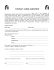### How to pass the RO6 Exam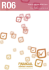### Manual Approach to Working in the Pelvic Region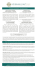### April 2015 Dear Applicant Thank you for the interest you have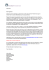### How to Increase Sales in a Slow Economy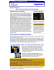### Document 6445035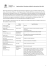### Final Spring 2015 Continuing Education Schedule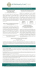### Hiroshi Itsuki, Asuka Takeuchi, Atsushi Fujita, Hitoshi Matsubara